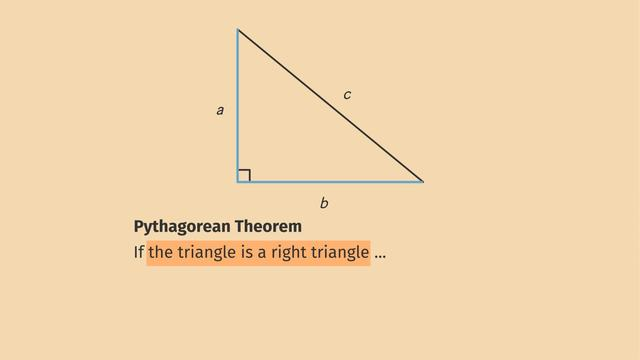# The Converse of the Pythagorean TheoremRating

Ø 3.7 / 3 ratings
The authorsChris S.

## Basics on the topicThe Converse of the Pythagorean Theorem

After this lesson, you will be able to prove the converse of the Pythagorean Theorem, i.e., if a2+b2=c2 in a triangle, then the triangle is right.

The lesson begins by teaching you that we can use indirect proof to prove the converse. By assuming the converse is NOT true, we can prove a contradiction, and conclude the converse IS true. It leads you to learn that assuming the converse is NOT true gives us acute and obtuse triangles we can use to prove a contradiction. It concludes with application of the converse to solve a problem.

Learn about the converse of the Pythagorean Theorem by helping Rana and her dragon decorate her dorm room!

This video includes key concepts, notation, and vocabulary such as the Pythagorean Theorem (given a right triangle, a2+b2=c2); converse of a theorem (formed by reversing the order of a theorem: the converse of p->q is q->p); indirect proof (assuming NOT x leads to a contradiction, which means x is true).

Before watching this video, you should already be familiar with the Pythagorean Theorem, isosceles triangles and the Isosceles Triangle Theorem (in an isosceles triangle, base angles are congruent).

After watching this video, you will be prepared to learn how to apply the Pythagorean Theorem and the converse to solve more complex problems, and how to use indirect proof to prove other theorems.

Common Core Standard(s) in focus: 8.G.B.6 A video intended for math students in the 8th grade Recommended for students who are 13 - 14 years old